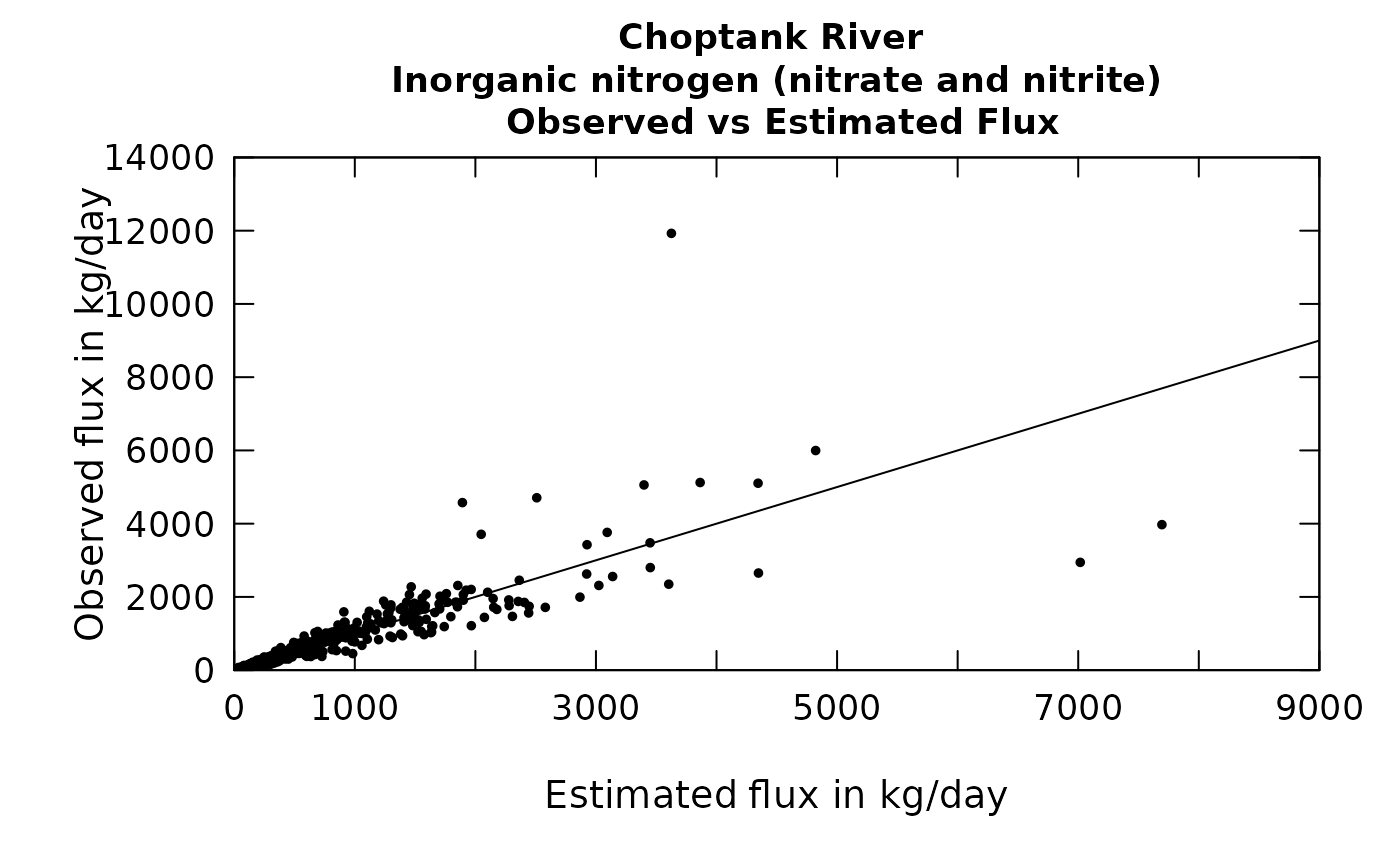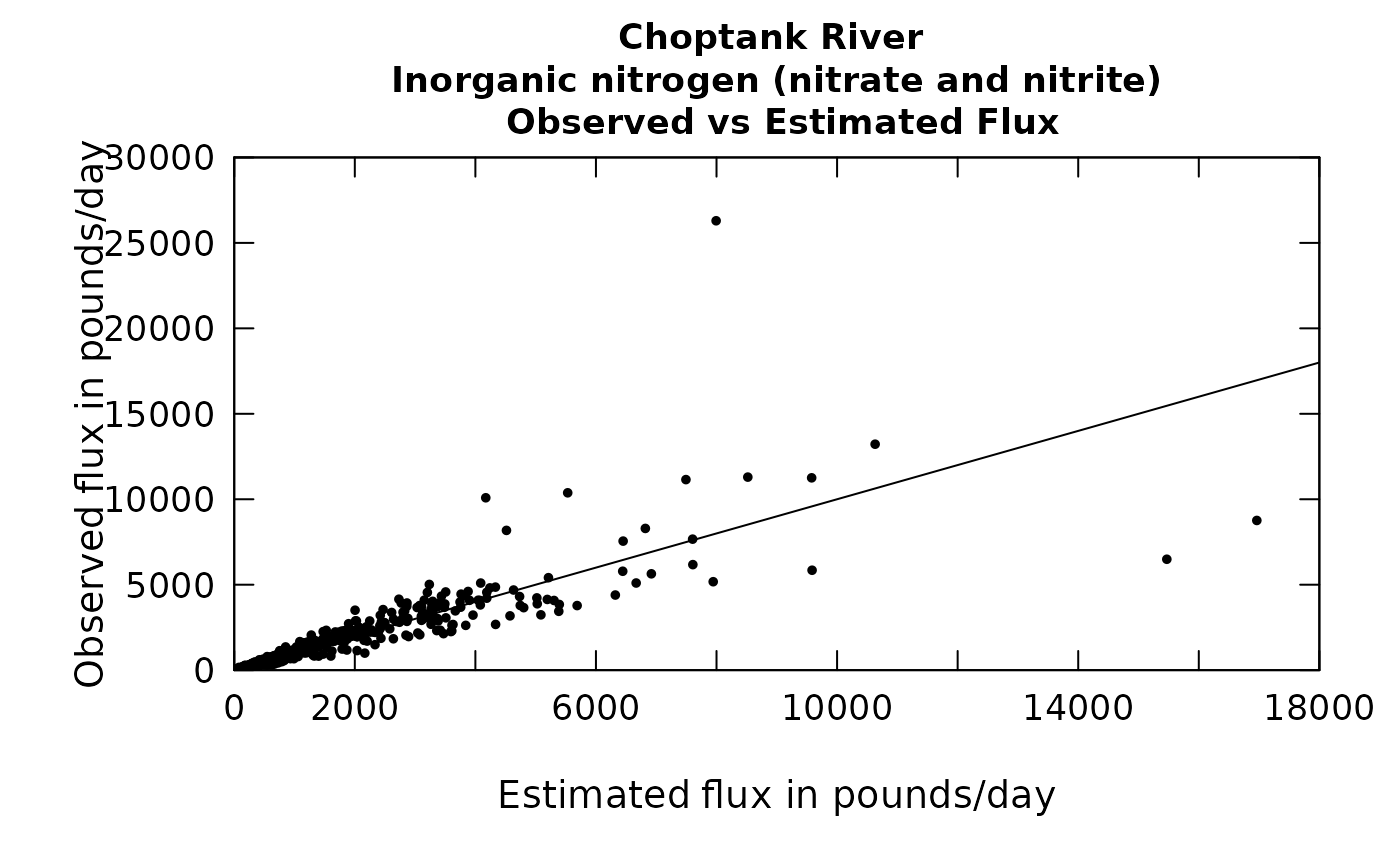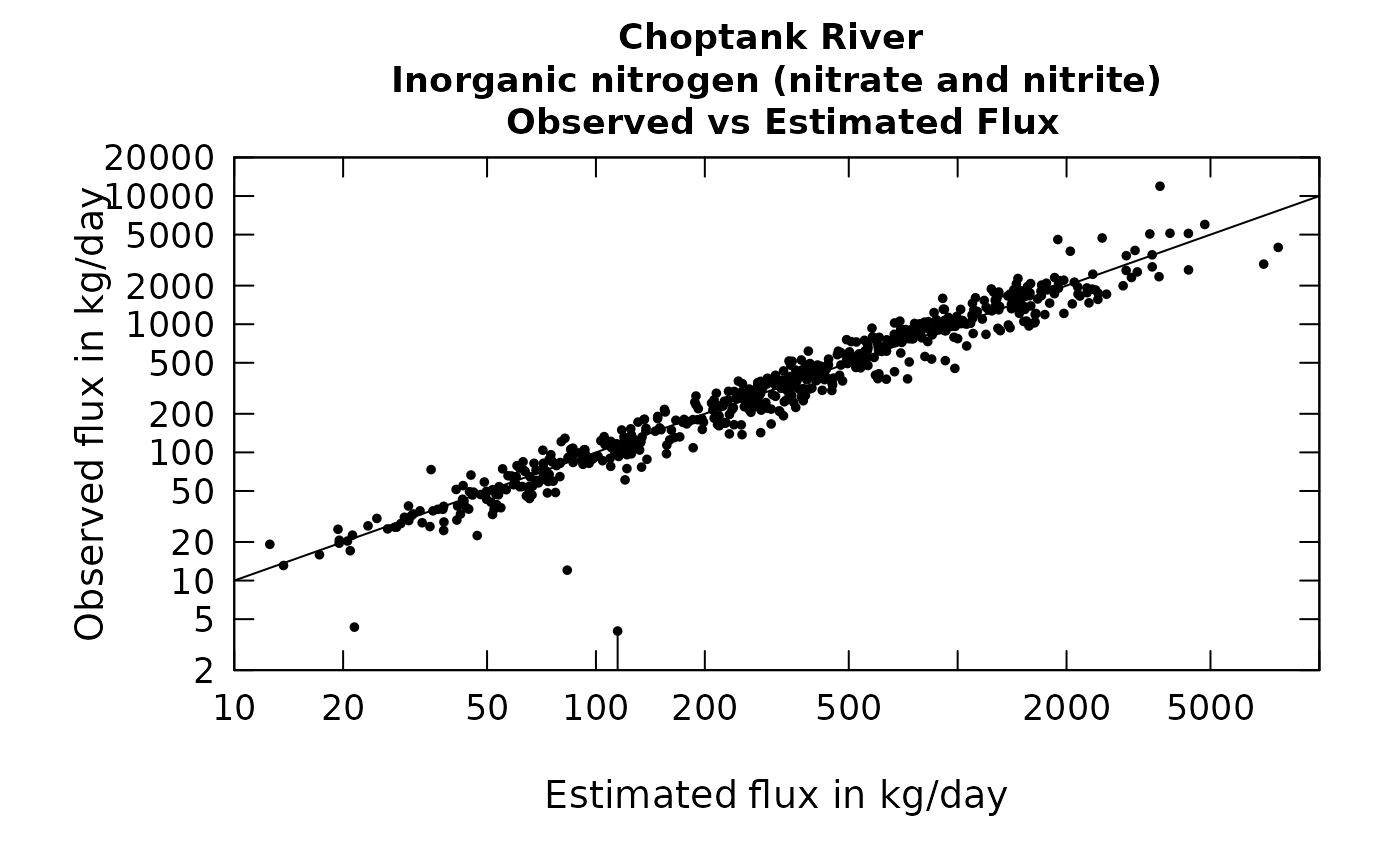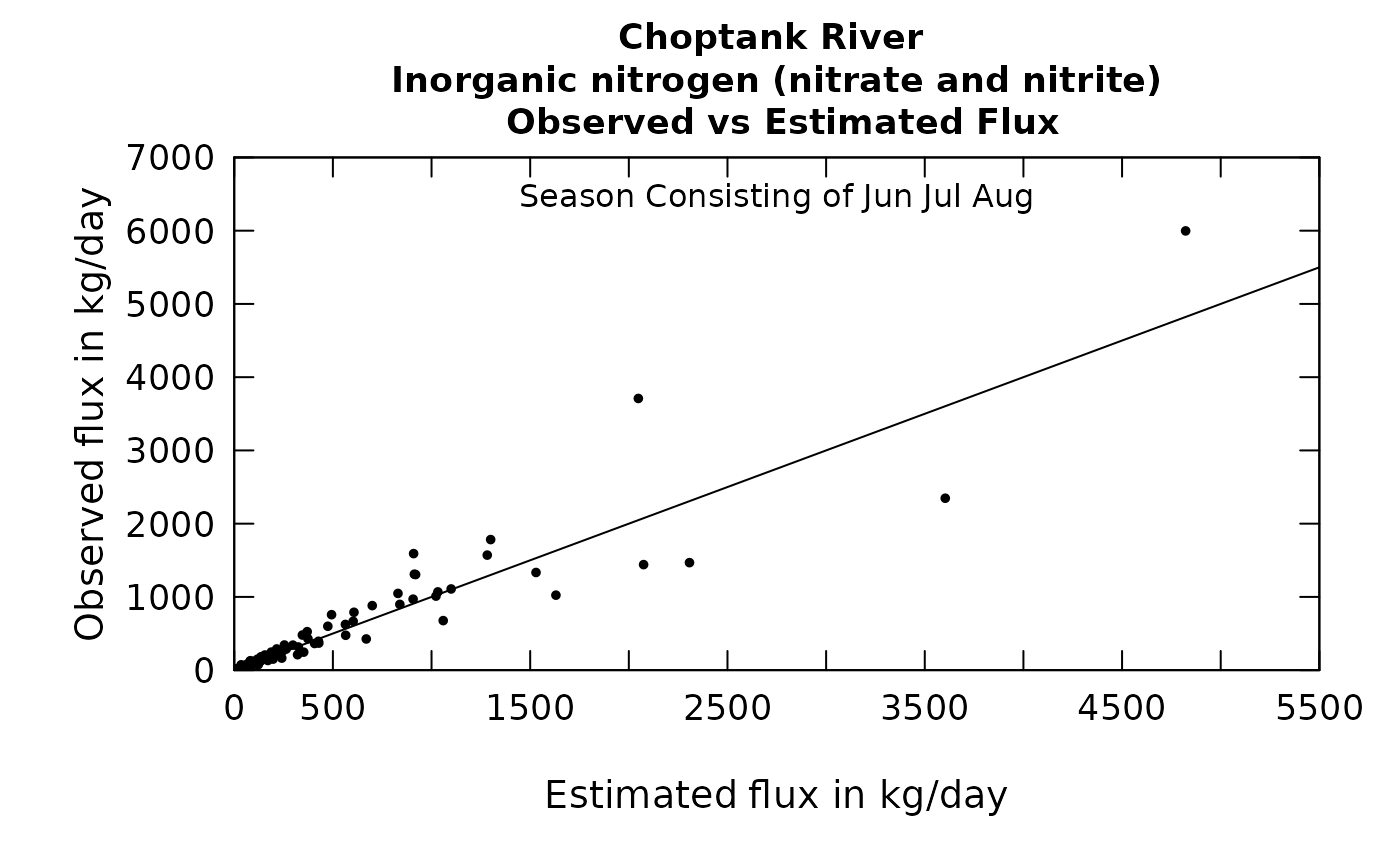Data come from named list, which contains a Sample dataframe with the sample data, and an INFO dataframe with metadata.

Although there are a lot of optional arguments to this function, most are set to a logical default.

## Usage

plotFluxPred(eList, fluxUnit = 3, fluxMax = NA, printTitle = TRUE,
oneToOneLine = TRUE, customPar = FALSE, col = "black", lwd = 1,
cex = 0.8, cex.axis = 1.1, cex.main = 1.1, tinyPlot = FALSE,
usgsStyle = FALSE, logScale = FALSE, randomCensored = FALSE, ...)

## Arguments

eList

named list with at least the Sample and INFO dataframes

fluxUnit

number representing entry in pre-defined fluxUnit class array. printFluxUnitCheatSheet

fluxMax

number specifying the maximum value to be used on the vertical axis, default is NA (which allows it to be set automatically by the data)

printTitle

logical variable if TRUE title is printed, if FALSE not printed (this is best for a multi-plot figure)

oneToOneLine

inserts 1:1 line

customPar

logical defaults to FALSE. If TRUE, par() should be set by user before calling this function (for example, adjusting margins with par(mar=c(5,5,5,5))). If customPar FALSE, EGRET chooses the best margins depending on tinyPlot.

col

color of points on plot, see ?par 'Color Specification'

lwd

number line width

cex

numerical value giving the amount by which plotting symbols should be magnified

cex.axis

magnification to be used for axis annotation relative to the current setting of cex

cex.main

magnification to be used for main titles relative to the current setting of cex

tinyPlot

logical variable if TRUE plot is designed to be small, if FALSE it is designed for page size, default is FALSE (not fully implemented yet)

usgsStyle

logical option to use USGS style guidelines. Setting this option to TRUE does NOT guarantee USGS compliance. It will only change automatically generated labels.

logScale

logical if TRUE x and y plotted in log axis

randomCensored

logical. Show censored values as randomized.

...

arbitrary graphical parameters that will be passed to genericEGRETDotPlot function (see ?par for options)

selectDays, genericEGRETDotPlot

## Examples

eList <- Choptank_eList
# Water year:
plotFluxPred(eList)plotFluxPred(eList, fluxUnit = 'poundsDay')plotFluxPred(eList, logScale=TRUE)# Graphs consisting of Jun-Aug
eList <- setPA(eList, paStart=6,paLong=3)
plotFluxPred(eList)